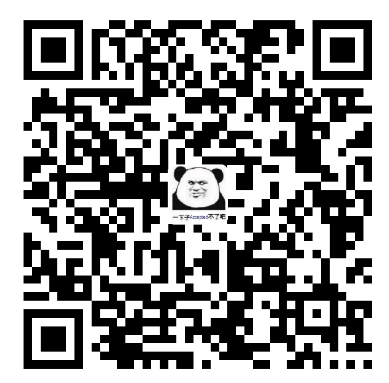### 信息的二进制编码

• 数值数据：包括无符号整数、带符号整数、浮点数
• 非数值数据：逻辑数（包含位串）、西文字符和汉字

• 真值：真正的值，也就是现实生活中带正负号的数
• 机器数：用0和1编码的计算机内部的0/1序列

### 定点数的编码表示

#### 原码

• 0的表示不唯一，不利于程序员编程
• 加减运算方式不统一
• 需要额外对符号位进行处理，不利于硬件设计
• 当a<b时，实现a-b比较困难

#### 补码

• 一个负数的补码等于模减去该负数的绝对值
• 对于某一确定的模而言，某数减去小于模的另一个数，总可以用该数加上另一个数负数的补码来实现，也就是可以用加法实现减法

$$10-4=10+\left( 12-4 \right) =10+8=6\left( mod12 \right)$$

$$9828-1928=9828+\left( 10^4-1928 \right) \\ =9828+8072=17900=7900\left( mod10^4 \right)$$

$$\left[ X \right] _{\text{补}}=2^n+X\left( -2^{n-1}\leqslant X<2^{n-1}, mod2^n \right)$$

#### 求真值的补码

123=127-4=01111111-100=01111011，所以-123=-01111011

$$\left[ 01111011 \right] _{\text{补}}=2^8+01111011=01111011\left( mod2^8 \right)$$

$$\left[ -01111011 \right] _{\text{补}}=2^8-01111011=100000000-01111011=11111111-0111011+1=10000101$$

#### 求补码的真值

• 符号为零，则为正数，数值部分相同
• 符号位1，则为负数，数值各位全部取反，末位加一（也可以用我上面说的简单方法）

### 移码

$$-8\left( +8 \right) ~0000 \\ -7\left( +8 \right) ~0001 \\ \cdots \\ 0\left( +8 \right) ~1000 \\ \cdots \\ +7\left( +8 \right) ~1111 \\$$

### 整数的表示

#### 带符号整数

• 补码运算系统是一种模运算系统，加、减运算统一，也就是说，可以使用加法来实现减法
• 0的表示唯一，方便使用
• 比原码多表示一个最小负数

• 由于0是unsigned类型的，所以-1也会被转换成unsigned，而其补码是32个1，被解析成无符号整数后就是$$2^{31}-1$$
• 第五个也是一样，右边的结果-2147483648的补码是首位1加上31个0，被解析成无符号数之后就是$$2^{31}$$
• 第六个例子中，2147483648U的补码同样也是首位的1加上31个0，但因为前面加上了一个（int）后，被转换成了一个带符号的整数，则在解释的时候就变成了$$-2^{31}$$

### 浮点数表示

• S：表示符号，0表示负数，1表示正数
• Exponent：表示阶码，也就是几次方
• 单精度规格化解码的范围为0000 0001到1111 1110（全零和全1用来表示特殊值）
• bias为127（单精度），1023（双精度）
• Significand：部分尾数，由于规格化尾数M是一个值在1-2之间的数，的最高位总是1，所以可以不表示出来，这样就可以节省一位。

#### 浮点数的表示范围

• 最大正数为：$$0.11\dots 1\times 2^{11\dots 1}=\left( 1-2^{-24} \right) \times 2^{127}$$
• 在这里解释一下，我们知道$$0.11\dots 1=1-0.00\dots 01$$，所以括号里的东西应该不难理解，阶码为什么是127呢？这是因为：移码=bias+指数本身，而$$\text{移码}=11111111=255$$，所以指数本身就等于255-bias = 255-128=127
• 最小正数位：$$0.10\dots 0\times 2^{00\dots 0}=\left( 1/2 \right) \times 2^{-128}$$
• 上面的补充看懂了下面的应该不难理解

#### 将机器数转换成真值：

$$\left( -1 \right) ^s\times \left( 1+Significand \right) \times 2^{\left( Exponent-127 \right)}$$

• 数符：1（负数）
• 阶：
• 阶码：001 1110 1 = 125
• 阶码的真值：阶码-bias = 125-127=-1
• 尾数：$$1+1\times 2^{-1}+1\times 2^{-2}+0\times 2^{-3}+1\times 2^{-4}+1\times 2^{-5}+\dots =1.75$$
• 真值：$$-1.75\times 2^{-2}=-0.4375$$

#### 将真值转换成机器数：

$$-12.75=-1100.11=-1.10011\times 2^3$$，阶为3，所以：

• 符号S = 1
• 阶码E = 127 + 3 = 128 + 2 = 10000010
• 部分尾数：100 1100 0000 0000 0000 0000

### 其他形式数的一些表示

0的表示：

• +0： 1 00000000 00000000000000000000000
• -0： 0 00000000 00000000000000000000000

• $$+\infty$$：0 11111111 00000000000000000000000
• $$-\infty$$： 1 11111111 00000000000000000000000

• 用指数部分全1，尾数部分非零来表示

### 非数值数据的编码表示

• 逻辑数据的编码表示
• 表示：用一位来表示，N位二进制数可表示N个逻辑数据
• 运算：按位进行，按位与之类的
• 识别：逻辑数据和数值数据在表示上一样，计算机依靠指令来识别
• 西文字符的编码表示
• 特点：只需对有限个字母和数字符号、标点符号等辅助字符编码，且所有字符总数不超过256个
• 表示：使用ASCII码表示
• 操作：字符串操作，比如比较之类的
• 汉字及国际字符的编码表示：
• 特点：数量多，表示麻烦
• 编码形式：一共有好几种：输入码、内码、字模点阵或轮廓描述

### 大端与小端

••